# Irreducible implies prime (PID)

## Statement

### Verbal statement

In a principal ideal domain, any irreducible element is a prime element.

### Symbolic statement

Let$R$ be a principal ideal domain and$p$ an irreducible element in$R$. Then, if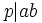$p|ab$, then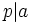$p|a$ or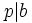$p|b$.

## Proof

Suppose$p|ab$ and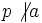$p \not | a$. We need to prove that$p|b$.

Then, the ideal generated by$p$ and$a$ is principal, and is generated by a factor of both$p$ and$a$. Since$p$ is irreducible, the only possibility for this is the whole ring. Thus: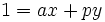$1 = ax + py$

for suitable choices of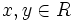$x,y \in R$.

Multiplying both sides by$b$, we get: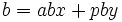$b = abx + pby$

Since$p|ab$,$p$ divides the entire right-hand-side, and hence$p|b$.